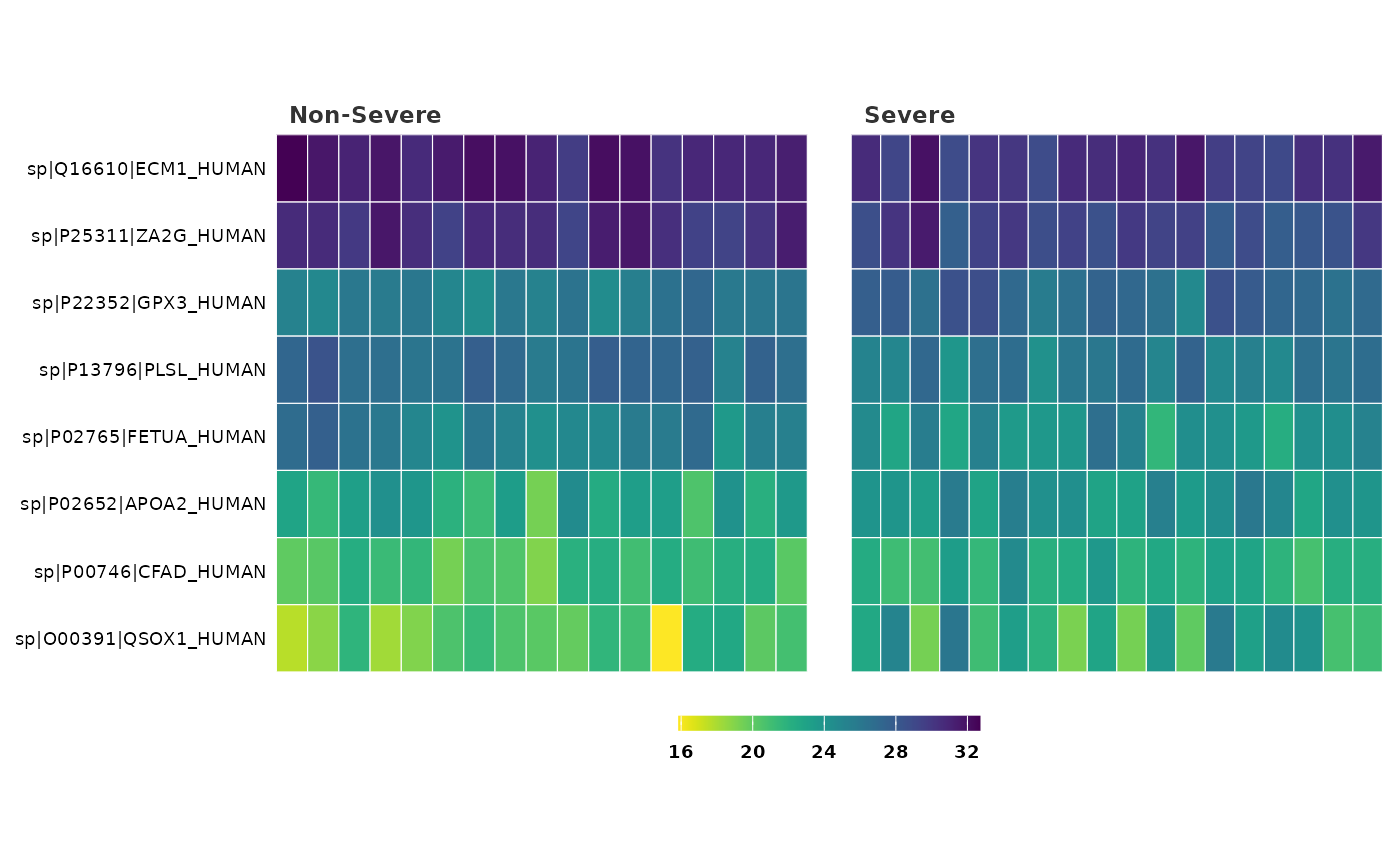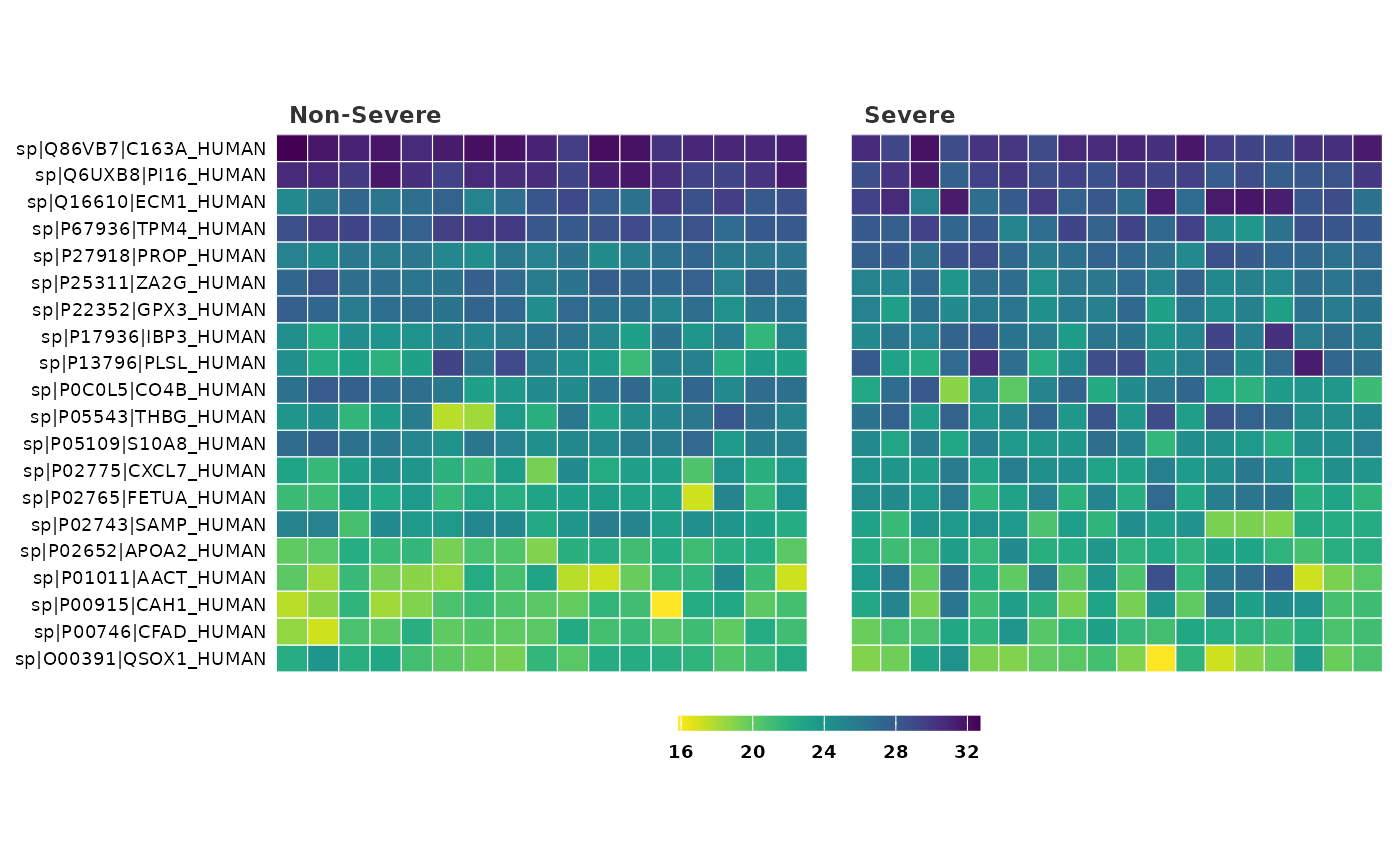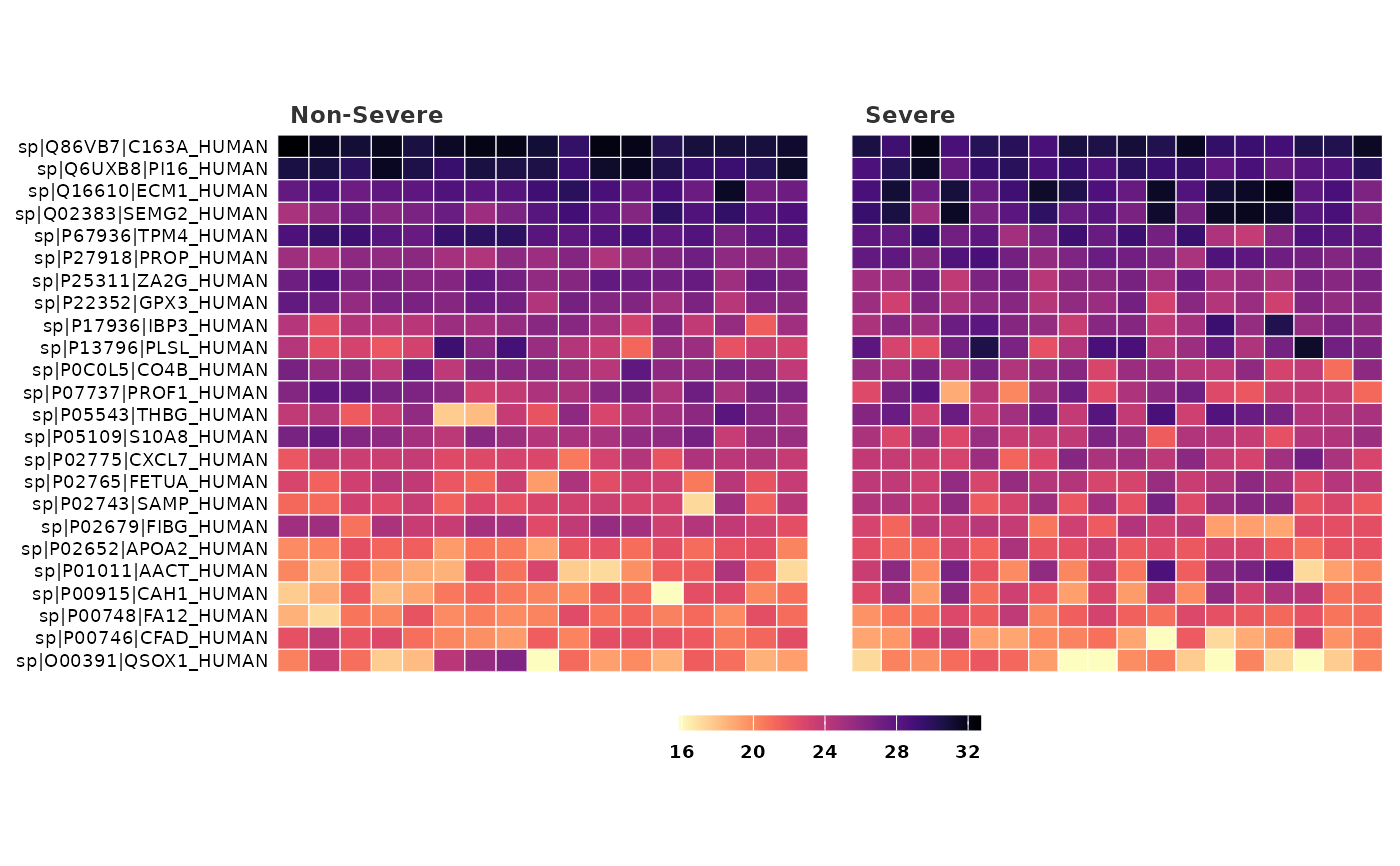This function generates a heatmap to visualize differentially expressed proteins between groups

## Usage

heatmap_de(
fit_df,
df,
cutoff = 0.05,
lfc = 1,
n_top = 20,
palette = "viridis",
text_size = 10,
save = FALSE,
file_path = NULL,
file_name = "HeatmapDE",
file_type = "pdf",
dpi = 80,
plot_height = 7,
plot_width = 7
)

## Arguments

fit_df

A fit_df object from performing find_dep.

df

The norm_df object or the imp_df object from which the fit_df object was obtained.

Method used for adjusting the p-values for multiple testing. Default is "BH".

cutoff

Cutoff value for p-values and adjusted p-values. Default is 0.05.

lfc

Minimum absolute log2-fold change to use as threshold for differential expression. Default is 1.

sig

Criteria to denote significance. Choices are "adjP" (default) for adjusted p-value or "P" for p-value.

n_top

Number of top hits to include in the heat map.

palette

Viridis color palette option for plots. Default is "viridis". See viridis for available options.

text_size

Text size for axis text, labels etc.

save

Logical. If TRUE saves a copy of the plot in the directory provided in file_path.

file_path

A string containing the directory path to save the file.

file_name

File name to save the plot. Default is "HeatmapDE."

file_type

File type to save the plot. Default is "pdf".

dpi

Plot resolution. Default is 80.

plot_height

Height of the plot. Default is 7.

plot_width

Width of the plot. Default is 7.

## Value

A ggplot2 plot object.

## Details

By default the tiles in the heatmap are reordered by intensity values along both axes (x axis = samples, y axis = proteins).

• find_dep

• topTable and lmFit functions from the limma package.

## Author

Chathurani Ranathunge

## Examples


## Build a heatmap of differentially expressed proteins using the provided
## example fit_df and norm_df data objects
heatmap_de(covid_fit_df, covid_norm_df)## Create a heatmap with P-value of 0.05 and log fold change of 1 as
## significance criteria.
heatmap_de(covid_fit_df, covid_norm_df, cutoff = 0.05, sig = "P")## Visualize the top 30 differentially expressed proteins in the heatmap and
## change the color palette
heatmap_de(covid_fit_df, covid_norm_df,
cutoff = 0.05, sig = "P", n_top = 30,
palette = "magma"
)